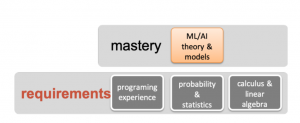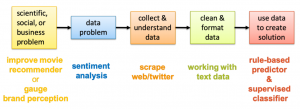## AI Education Matters: A first introduction to modelling and learning using the data science workflow

January 21, 2020AI Education Matters is a regular feature in the AI Matters newsletter. Here, Marion Neumann explains the teaching methods she uses to expose students to the ideas and working principles of AI technology.

## Introduction

Traditionally artificial intelligence (AI) and machine learning (ML) courses are taught at the senior and graduate level in higher-education computer science curricula following the mastery learning strategy, cf. Figure 1.This makes sense, since most AI and ML models and the theory behind them require a substantial understanding of probability and statistics, as well as advanced calculus and matrix algebra. To understand Logistic Regression as a probabilistic classifier performing maximum-likelihood or maximum-a-posteriori estimation, for example, students need to understand joint and conditional probability distributions. In order to derive the back propagation algorithm to train Neural Networks students need to understand partial derivatives and inner and outer tensor products. These are just two of many examples where substantial mathematical background – typically taught at the junior level in a computer science major program – is required. With AI and ML algorithms being used more widely by enterprises across domains, as well as, in applications and services we use in our daily lives, it makes sense to raise awareness about what AI is, what it can and cannot do, and how it is used to solve problems to a broader audience. Very much in the same spirit as the “CS for all” idea (https://www.csforall.org), we have to extend our curricula to include introductory courses to AI and ML on the early undergraduate level (or even in highschool) to expose students to the ideas and working principles of AI technology. One way to achieve this is to introduce the principles of working with data, modeling, and learning through the data science workflow.

## Exposure First

Following the exposure – interest – mastery paradigm as illustrated in Figure 2, we propose to gently introduce AI/ML concepts focusing on example applications rather than computational problems by incorporating course modules into introductory CS courses or design an entire course early on the curriculum. The goal of such intro-level modules or courses is to expose students to AI/ML problems and introduce basic techniques to solve them without relying on the computational and mathematical prerequisite knowledge. More concretely, the module or course may be designed as combined lecture and lab sessions, where a new topic is introduced in a lecture unit followed by a lab session, where students get to know a problem in the context of an application, explore a solution method, and tackle a potentially open-ended question about evaluation procedures, benefits and challenges of the approach, or implications and ethical considerations when using such methods in the real world in a group discussion. Lab sessions should be designed carefully focusing on the understanding of the data, the problem, and the results instead of model implementation. We will introduce two such lab assignments implemented in Python and Jupyter notebooks in the next section.The main aim of our assignments is to engage the students’ interest to acquire the prerequisite knowledge in order to move forward and gain a deeper understating of specific AI and ML techniques. Since we propose these units for a course that is taught very early in the CS curriculum, we face the challenge that students do not have a lot of programming experience nor a deep understanding of data structures and algorithms. Therefore, we developed the lab assignments using Jupyter notebooks which nicely combine illustrative instructions and executable starter code.

After having worked though the data science workflow using illustrative applications that are both easy to understand and relevant in the real-world, our hope is that students develop the motivation to study traditional prerequisite classes for AI and ML courses like probability and statistics, matrix/linear algebra, and algorithm analysis perceiving them useful to master AI/ML instead of a nuisance.

## Two Model AI Assignments

### Introduction to Python for Data Science

We provide an interactive guided lab to introduce Python for data science (DS), which can also be used for any course that introduces modeling and learning using Python, such as introduction to AI or ML courses. We provide two Jupyter notebooks, one introducing the basics of Python and the other the DS workflow using the Iris dataset (https://archive.ics.uci.edu/ml/datasets/Iris). We interactively introduce the use of expressions, variables, strings, printing, lists, dictionaries, control flow, and functions in Python to students that are already familiar with a programming language from an introductory CS course. The second lab aims at motivating students to acquire skills such as using statistics to model and analyze data, knowing how to design and use algorithms to store, process, and visualize data, while not forgetting the importance of domain expertise. We begin by establishing the example problem to be studied based on the Iris dataset. The next step is to acquire and process the data, where students practice how to load data and process strings into numeric arrays using numpy. Then, we explain different plotting methods such as box plots, histograms, and scatter plots for data exploration leveraging matplotlib. Finally, we split the data into training and test set, build a model, use it for predictions, and evaluate the results using sklearn. The main learning objectives are to get to know and practice Python in the context of a realistic data science and machine learning application.

### Introducing the Data Science Workflow using Sentiment Analysis

The second interactive lab guides students through a basic data science workflow by exploring sentiment analysis. The data science workflow along with the example sentiment analysis application is depicted in Figure 3. The lab assignment focuses on introducing the machinery using a given dataset of movie reviews. We further provide a follow-up homework assignment reiterating some of the steps and highlighting data acquisition and exploration with Twitter data. After introducing sentiment analysis, we explain a simple rule-based approach to predict the sentiment of textual reviews using three handcrafted examples. This introduction shows simple means to preprocess text data and exemplifies the use of lists of positive and negative expressions to compute a sentiment score. Then students will implement the approach to predict the sentiment of movie reviews and evaluate the results. The lab concludes with a discussion of the limitations of the rule-based approach and a quick introduction to sentiment classification via machine learning. The homework assignment reiterates over the process of building and analyzing a sentiment predictor with the focus on collecting and preprocessing their own dataset scraped from Twitter using the python-twitter API. The main learning objective of this activity is getting to know the inference problem and walking through the entire data science workflow to tackle it. Since the module only requires minimal programming background it is an ideal precursor to introducing machine learning in an AI, ML, or DS course. It may also be used in a introduction to Python course as a module focusing on using libraries and APIs.Figure 3: Data science workflow using sentiment analysis as an example application.

## Our Experiences

We incorporated both lab assignments into our “Introduction to Data Science” course for sophomore students at Washington University in St. Louis. One of the challenges we faced was that our students had different levels of Python experience, from no experience at all (51%) over some experience (36%) to quite proficient (13%). This led to a large variance in the times needed to complete the labs. To deal with this issue we propose to add some optional challenge problems to the assignment that are not required for the homework or will be introduced later in the course. Another challenge was that some students preferred to work in groups where others did the labs on their own. However, both strategies can result in slower or faster pace given the students’ working style, group composition, and amount of group discussion. Unfortunately, there is no unified way to tackle this issue, however, we believe that students should be encouraged to work in teams for the lab assignments, whereas homework assignments should be worked on individually. This way both teamwork and communication skills as well as knowledge retention are facilitated.

Both labs were perceived as useful by our students. 97% answered Yes to the question “Did you like the lab.” for the introduction to Python lab and 81% for the sentiment analysis lab. The most common reasons stated by students that didn’t like the second lab were that they where overwhelmed by unfamiliar code and that it was too long. From the students’ answers to our quiz and exam questions we can also confirm that they understand basic Python processes to handle data, implement and apply simple learning models, and visualize and interpret their results.

## Pedagogical Resources

In addition to Jupyter notebooks constituting the lab and homework assignments, we developed lecture materials in form of slides and worksheets for each module. The first lecture covers an introduction to data science and machine learning, and the second one introduces sentiment analysis, text processing, and classification respectively. The slides are interactive with gaps to be filled in by the instructor during the lectures and the worksheets contain in-class activities for students to engage with the presented materials. Those resources are available from the authors upon request.

Useful textbooks that specifically focus on introducing data science topics and techniques are:
• Python Data Science Handbook VanderPlas (2016) introduces essential tools and libraries such as Jupyter notebooks, numpy, pandas, scikit-learn, and matplotlib for working with data.
• Data Science from Scratch Grus (2019) focuses on implementing learning algorithms and data processing routines from scratch.
• Data Science for Business Provost and Fawcett (2013) showcases interesting realworld use cases and emphasizes data analytic thinking while not being too technical.

The first two books focus on implementations in Python, whereas the third one details concepts and techniques without code examples.

### References

• Grus, J. (2019). Data science from scratch: first principles with python. O’Reilly Media.
• Provost, F., & Fawcett, T. (2013). Data science for business: What you need to know about data mining and dataanalytic thinking. ” O’Reilly Media, Inc.”.
• VanderPlas, J. (2016). Python data science handbook: essential tools for working with data. ” O’Reilly Media, Inc.”
AI Matters is the blog and newsletter of the ACM Special Interest Group on Artificial Intelligence... read more

Marion Neumann is a Senior Lecturer at Washington University in St. Louis and the SIGAI diversity officer... read more